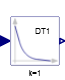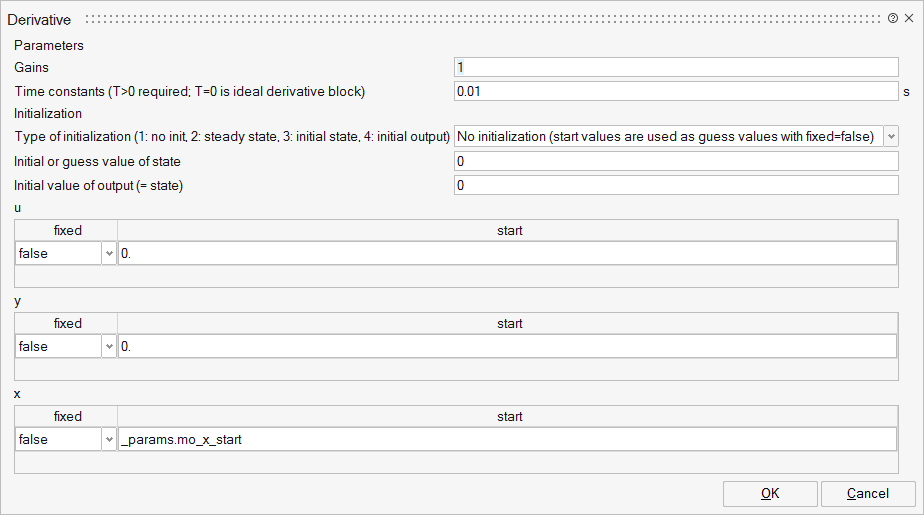# Derivative

Approximated derivative block## Library

Blocks/Continuous

## Description

This blocks defines the transfer function between the input u and the output y as approximated derivative:

```             k * s
y = ------------ * u
T * s + 1
```

If you would like to be able to change easily between different transfer functions (FirstOrder, SecondOrder, ... ) by changing parameters, use the general block TransferFunction instead and model a derivative block with parameters
b = {k,0}, a = {T, 1}.

If k=0, the block reduces to y=0.

## ParametersNameLabelDescriptionData TypeValid Values

mo_k

Gains

Scalar

mo_T

Time constants (T&gt;0 required; T=0 is ideal derivative block)

Scalar

mo_initType

Type of initialization (1: no init, 2: steady state, 3: initial state, 4: initial output)

Structure

mo_initType/choice1

No initialization (start values are used as guess values with fixed=false)

Number

0
1

mo_initType/choice2

Steady state initialization (derivatives of states are zero)

Number

0
1

mo_initType/choice3

Initialization with initial states

Number

0
1

mo_initType/choice4

Initialization with initial outputs (and steady state of the states if possible)

Number

0
1

mo_x_start

Initial or guess value of state

Scalar

mo_y_start

Initial value of output (= state)

Scalar

mo_zeroGain

zeroGain

Modelica default value: abs(k) < Modelica.Constants.eps

Scalar

true
false

mo_u

u

Structure

mo_u/fixed

fixed

Cell of scalars

true
false

mo_u/start

start

Cell of scalars

mo_y

y

Structure

mo_y/fixed

fixed

Cell of scalars

true
false

mo_y/start

start

Cell of scalars

mo_x

x

Structure

mo_x/fixed

fixed

Cell of scalars

true
false

mo_x/start

start

Cell of scalars

## Ports

NameTypeDescriptionIO TypeNumber

u

implicit

Connector of Real input signal

input

1

y

implicit

Connector of Real output signal

output

1